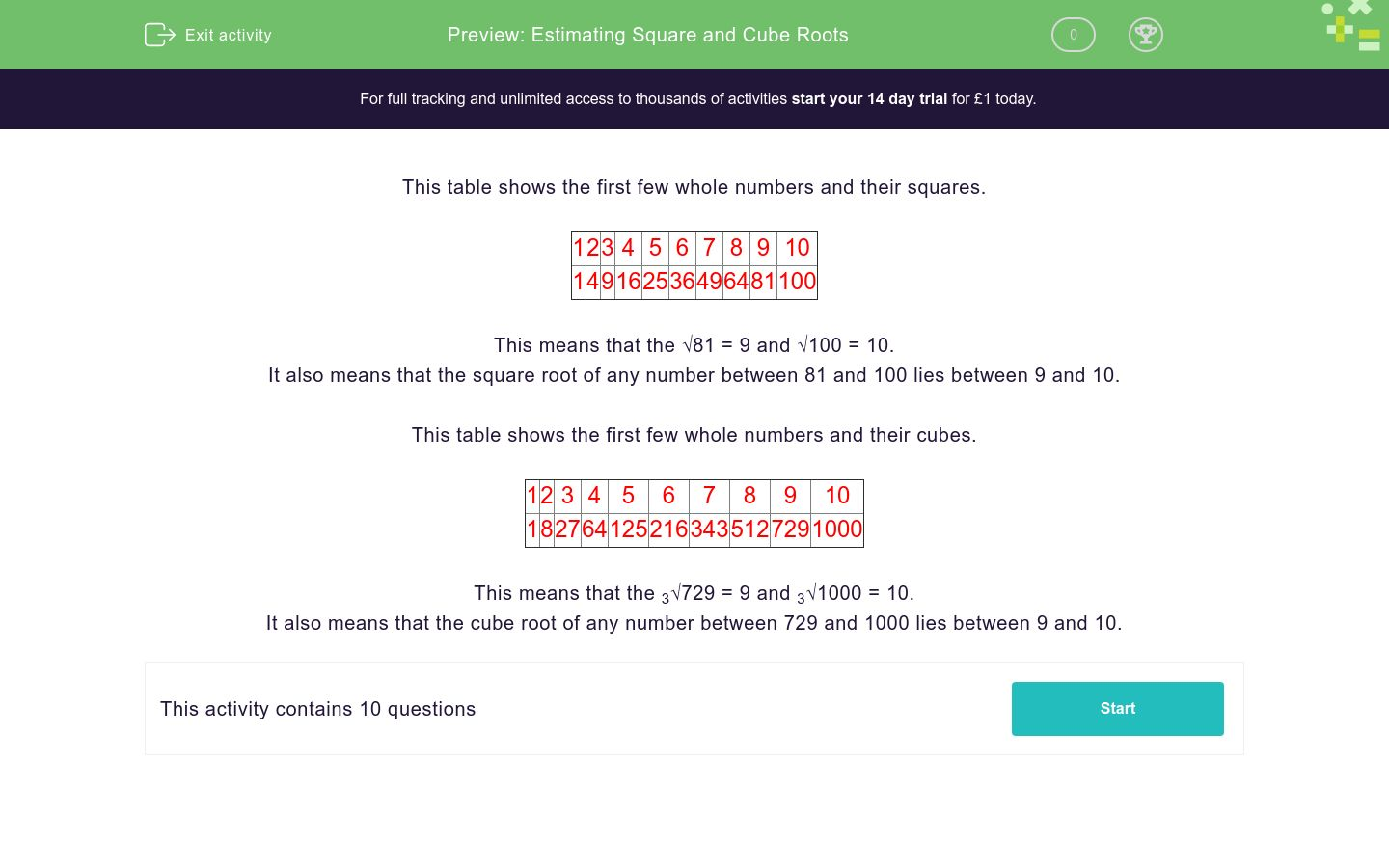# Estimating Square and Cube Roots

In this worksheet, students estimate square roots and cube roots.Key stage:  KS 3

Curriculum topic:  Number

Curriculum subtopic:  Understand Integer Powers/Real Roots

Difficulty level:### QUESTION 1 of 10

This table shows the first few whole numbers and their squares.

 1 2 3 4 5 6 7 8 9 10 1 4 9 16 25 36 49 64 81 100

This means that the √81 = 9 and √100 = 10.

It also means that the square root of any number between 81 and 100 lies between 9 and 10.

This table shows the first few whole numbers and their cubes.

 1 2 3 4 5 6 7 8 9 10 1 8 27 64 125 216 343 512 729 1000

This means that the 3√729 = 9 and 3√1000 = 10.

It also means that the cube root of any number between 729 and 1000 lies between 9 and 10.

Between which two numbers does the following square root lie?

√10

3 and 4

2 and 3

7 and 8

Between which two numbers does the following square root lie?

√30

5 and 6

2 and 3

3 and 4

Between which two numbers does the following cube root lie?

3√62

7 and 8

2 and 3

3 and 4

Between which two numbers does the following square root lie?

√50

7 and 8

2 and 3

3 and 4

Between which two numbers does the following cube root lie?

3√50

3 and 4

6 and 7

7 and 8

Between which two numbers does the following square root lie?

√40

5 and 6

6 and 7

7 and 8

Between which two numbers does the following cube root lie?

3√420

5 and 6

6 and 7

7 and 8

Between which two numbers does the following cube root lie?

3√999

7 and 8

8 and 9

9 and 10

Between which two numbers does the following square root lie?

√65

4 and 5

8 and 9

9 and 10

Between which two numbers does the following cube root lie?

3√65

4 and 5

8 and 9

9 and 10

• Question 1

Between which two numbers does the following square root lie?

√10

3 and 4
• Question 2

Between which two numbers does the following square root lie?

√30

5 and 6
• Question 3

Between which two numbers does the following cube root lie?

3√62

3 and 4
• Question 4

Between which two numbers does the following square root lie?

√50

7 and 8
• Question 5

Between which two numbers does the following cube root lie?

3√50

3 and 4
• Question 6

Between which two numbers does the following square root lie?

√40

6 and 7
• Question 7

Between which two numbers does the following cube root lie?

3√420

7 and 8
• Question 8

Between which two numbers does the following cube root lie?

3√999

9 and 10
• Question 9

Between which two numbers does the following square root lie?

√65

8 and 9
• Question 10

Between which two numbers does the following cube root lie?

3√65